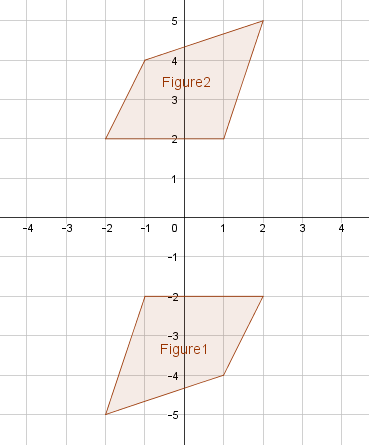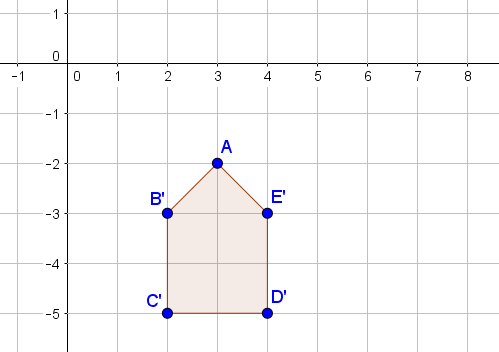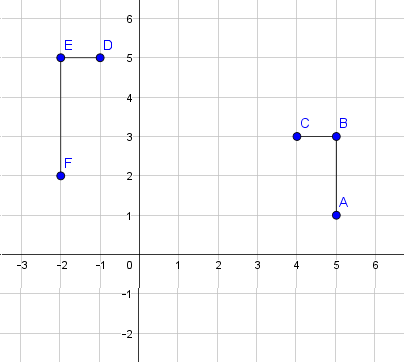# Transformations and Angle Relationships

## Objective

Determine and informally prove or disprove if two figures are similar or congruent using transformations.

## Common Core Standards

### Core Standards

?

• 8.G.A.2 — Understand that a two-dimensional figure is congruent to another if the second can be obtained from the first by a sequence of rotations, reflections, and translations; given two congruent figures, describe a sequence that exhibits the congruence between them.

• 8.G.A.4 — Understand that a two-dimensional figure is similar to another if the second can be obtained from the first by a sequence of rotations, reflections, translations, and dilations; given two similar two-dimensional figures, describe a sequence that exhibits the similarity between them.

?

• 7.G.A.1

• 7.RP.A.2

## Criteria for Success

?

1. Determine if two figures are similar and if so, describe the sequence of dilations and transformations that maps one to the other.
2. Determine if two figures are congruent, and if so, describe the sequence of rigid transformations that maps one to the other.

## Tips for Teachers

?

• This lesson continues from Lesson 13 in that all transformations (dilations and rigid motions) are brought together.
• This lesson can be used as a flex or review day depending on what students need.
• The following materials are useful for this lesson: patty paper (or transparency paper), graph paper, and rulers.

#### Fishtank Plus

• Problem Set
• Student Handout Editor
• Vocabulary Package

## Anchor Problems

?

### Problem 1

Two figures are shown below.For each statement below, determine if it is true or false. Explain your reasoning.

1. A reflection over the $x$-axis followed by a translation to the left will transform Figure 1 to Figure 2, making the two figures congruent.
2. A rotation of ${180^{\circ}}$ about the origin will transform Figure 1 to Figure 2, making the two figures congruent.
3. A dilation from the origin and a translation up will transform Figure 1 to Figure 2, making the two figures similar.
4. A reflection across the $y$-axis followed by a reflection across the $y$-axis will transform Figure 1 to Figure 2, making the two figures congruent.
5. There is no sequence that will transform Figure 1 to Figure 2, making the two figures neither congruent nor similar.

### Problem 2

The pentagon shown below is the resulting image of pentagon ${ABCDE}$ after it was dilated from point $A$ by a scale factor of ${\frac{1}{2}}$ and then rotated clockwise ${90^{\circ}}$ about point $A$

What are the original coordinates of pentagon ${ABCDE}$?## Problem Set

?The following resources include problems and activities aligned to the objective of the lesson that can be used to create your own problem set.

• Multiple-choice options are good problems to engage students in evaluating multiple possible answer choices. Examples to include:
• Given similar or congruent figures, select the correct description of the transformation sequence.
• Multiple-select items about properties of transformations where there is more than one correct answer.